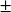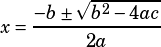2x^2 – 3x – 7 = 0

If c and d are the two solutions of the quadratic equation above, what is the value of c + d?

Is there a smarter/quicker way to solve this than the quadratic formula?

Yes and no. Which is to say, you should use the quadratic formula, but you don’t have to take it all the way. The two solutions you get when you do the quadratic formula come from thein. But in this case, since we’re adding the two solutions, everything after thewill cancel out! Let’s sayandare the “plus” and “minus” solutions, respectively. Then we can say:That’s a lot easier to work with, right?aarhin10 says:aarhin10 says: